# Using Operations

The following table shows an example of how to use the above operations to create and manipulate a queue. It assumes the steps are performed sequentially and the result of the operation is shown.

Step Operation Effect
1 Constructor Creates an empty queue of capacity 3.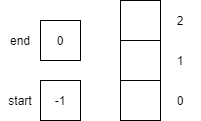2 `isFull()` Returns `false` since `start` is not equal to `end`3 `isEmpty()` Returns `true` since `start` is equal to `-1`.4 `enqueue(1)` Places item `1` onto queue at the end and increments `end` by 1.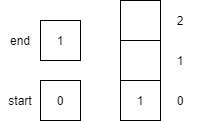5 `enqueue(2)` Places item `2` onto queue at the end and increments `end` by 1.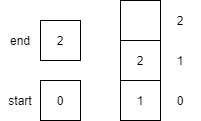6 `enqueue(3)` Places item `3` onto queue at the end and sets `end` to `end+1` modulo the size of the array (3). The result of the operation is `0`, thus `end` is set to `0`.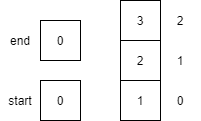7 `peek()` Returns the item `1` on the `start` of the queue but does not remove the item from the queue. `start` and `end` are unaffected by peek.8 `isFull()` Returns `true` since `start` is equal to `end`9 `dequeue()` Returns the item `1` from the start of the queue. `start` is incremented by 1, effectively removing `1` from the queue.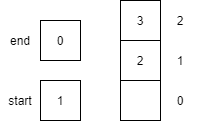10 `dequeue()` Returns the item `2` from the start of the queue. `start` is incremented by 1, effectively removing `2` from the queue.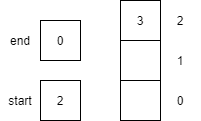11 `enqueue(4)` Places item `4` onto queue at the end and increments `end`.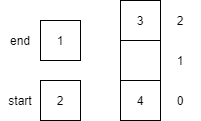12 `dequeue()` Returns the item `3` from the start of the queue. `start` is set to `start+1` modulo the size of the array (3). The result of the operation is `0`, thus `start` is set to `0`.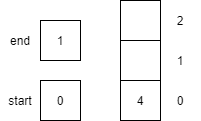13 `dequeue()` Returns the item `4` from the start of the queue. `start` is incremented by 1, and since `start == end`, they are both reset to `-1` and `0` respectively.14 `isEmpty()` Returns `true` since `start` is equal to -1.